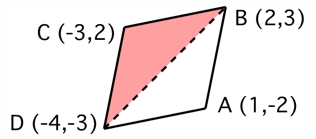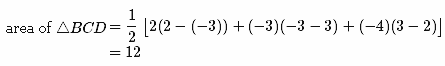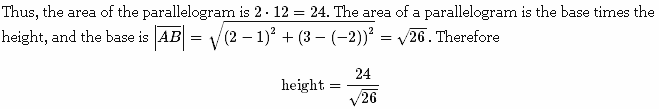Request a call back

while boarding an aeroplane a passenger got hurt .the pilot showing promptness and concern made arrangements to hospitalise the injured and so the plane started late by 30 minutes.to reach the destination,1500 km away the pilot increased the speed by 100 km/h.find the original speed
Asked by rosetta | 09 Mar, 2013, 09:05: PMExpert Answer
1.

We are given that the distance = 1500 km
Let the time taken by the aeroplane at its usual speed be T hours and its usual speed be S kmph
Case 1(usual speed):
T = D/S = 1500/S ....(1)
Case 2(speed was increased):
Speed = (S+100) kmph
As the aeroplane left half an hour late and still reached the destination in time, time taken = (T - 1/2) hours
Therefore we have:
T - 1/2 = D/(S+100) = 1500/(S+100)
From (1):
1500/S - 1/2 = 1500/(S+100)
⇒1500/S - 1500/(S+100) = 1/2
⇒1/S - 1/(S+100) = 1/3000
⇒(S+100-S)3000 = S(S+100)
⇒300000 = S^2 + 100S
⇒S^2 + 100S - 300000 = 0
⇒S^2+600S-500S-300000 = 0
⇒S(S+600)-500(S-600) = 0
⇒(S-500)(S+600) = 0
Therefore, either S = 500 or S = -600. Since speed of the aeroplane cannot be negative, we reject S = -600. Therefore, the usual speed of the aeroplane is 500 kmph.

2.

We know that the diagonal of a parallelogram divides it into 2 triangles of equal areas.Answered by | 11 Mar, 2013, 10:19: AM

## Concept Videos

CBSE 10 - Maths
Asked by varma.renu9481 | 25 Jan, 2023, 04:11: PMANSWERED BY EXPERT
CBSE 10 - Maths
Asked by yogitamanojkumar | 09 Jan, 2023, 04:01: PMANSWERED BY EXPERT
CBSE 10 - Maths
hiAsked by chaudahryanshika | 03 Jan, 2023, 10:04: AMANSWERED BY EXPERT
CBSE 10 - Maths
Asked by nk.neetuhome | 18 Nov, 2022, 03:43: PMANSWERED BY EXPERT
CBSE 10 - Maths
Asked by Subuhiansari67 | 28 Sep, 2022, 10:11: PMANSWERED BY EXPERT
CBSE 10 - Maths
Asked by devchauhan25007 | 17 Jul, 2022, 04:29: PMANSWERED BY EXPERT
CBSE 10 - Maths
Asked by rajendrame089 | 28 Jun, 2022, 07:09: AMANSWERED BY EXPERT
CBSE 10 - Maths
Asked by gurjaeharsh | 16 Jun, 2022, 01:11: PMANSWERED BY EXPERT
CBSE 10 - MathsANSWERED BY EXPERTANSWERED BY EXPERT